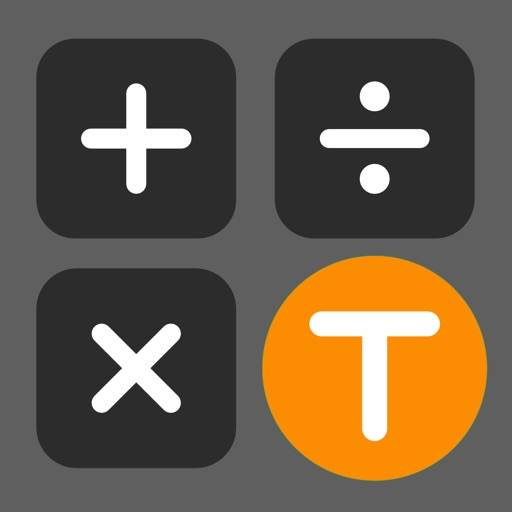# Twin-Calc - Two calculators are in One App\$3.99

Company: Hiroyuki Ueda

App Genre: Utilities

Ratings: 0.00

Version: 2.5.2

Released: Apr 16, 2022

Updated Sep 14, 2022

Twin-Calc is a utility app developed by Hiroyuki Ueda and available for download on the iOS App Store and Android Play Store. The app is compatible with iOS 13.0 or later and requires 689.00kB of free space to install.

It is a simple and easy-to-use calculator app that combines two calculators into one.

If you tilt the screen to the landscape position, another calculator will appear next to it.

In addition to being able to perform separate calculations with each calculator, the calculation results can be copied and pasted to each other with a single touch, so you can perform different calculations during the calculation, and you can use it more conveniently than you can imagine.

There is also a free version that displays ads. Please try it first.
https://apps.apple.com/jp/app/t-calc-lite/id1630026837?l=jp

【feature】
1. You can copy and paste the calculation results between two calculators.
If you tilt the screen landscape position, the secod calculators will be displayed.
The result calculated by one calculator can be input to the other calculator with one touch.
You can perform another calculation in the middle of the calculation and input the result to the other calculator with one touch.

・ When you press the "→" key, the calculation results of the left calculator will be input to the right calculator.
・ When you press the "←" key, the calculation result of the right calculator will be input to the left calculator.

Example of use
For example, if the meal fee is 3,000 yen per person, the drink fee is 500 yen per person, and the room fee is 3,500 yen per room for three people.

1) First, calculate the meal + drink fee with the calculator on the right.
Where you entered 3000 + 500+

2) Calculate the room charge per person with the calculator on the left
3600/3 = 1200

3) Press the "→" key and paste the calculation result "1200" of the left calculator into the right calculator.
3000 + 500 + 1200

4) The total cost per person can be calculated.
3000 + 500 + 1200 = 4700

2. Addition, subtraction, multiplication and division are possible.
・ Calculations are performed first for multiplication and division, and later for addition and subtraction.

3. Display the calculation formula
Since the entered calculation formula is displayed, you can easily check the numerical values and operators.

Limitations
・ The answer can be calculated up to 9 digits and a minimum of 0.000000001 (including the code).
・ When the value is divided by 0, it will be ∞ correctly, but it will be displayed as "0" on this calculator.

Note
Free of charge version can be download from
https://apps.apple.com/jp/app/t-calc-lite/id1630026837?l=en

Need help? Join our Twin-Calc forum to get help, ask questions, and discuss the app with other users from all over the world.

Twin-Calc can also be downloaded from the following language pages:
Germany